[BACK]
 Computers, Materials & ContinuaDOI:10.32604/cmc.2022.022809Article

Fruit Image Classification Using Deep Learning

1Department of Computer Science, Guru Arjan Dev Khalsa College Chohla Sahib, 143408, Punjab, India
2Al-Nahrain University, Al-Nahrain Nano-Renewable Energy Research Center, Baghdad, 964, Iraq
3Department of Computer Science, College of Computer and Information Systems, Umm Al-Qura University, Makkah, 21955, Saudi Arabia
4Department of Information Technology, College of Computers and Information Technology, Taif University, Taif, 21944, Saudi Arabia
5Department of Computer Engineering, College of Computers and Information Technology, Taif University, Taif, 21944, Saudi Arabia
*Corresponding Author: Harmandeep Singh Gill. Email: profhdsgill@gmail.com
Received: 19 August 2021; Accepted: 10 November 2021

Abstract: Fruit classification is found to be one of the rising fields in computer and machine vision. Many deep learning-based procedures worked out so far to classify images may have some ill-posed issues. The performance of the classification scheme depends on the range of captured images, the volume of features, types of characters, choice of features from extracted features, and type of classifiers used. This paper aims to propose a novel deep learning approach consisting of Convolution Neural Network (CNN), Recurrent Neural Network (RNN), and Long Short-Term Memory (LSTM) application to classify the fruit images. Classification accuracy depends on the extracted and selected optimal features. Deep learning applications CNN, RNN, and LSTM were collectively involved to classify the fruits. CNN is used to extract the image features. RNN is used to select the extracted optimal features and LSTM is used to classify the fruits based on extracted and selected images features by CNN and RNN. Empirical study shows the supremacy of proposed over existing Support Vector Machine (SVM), Feed-forward Neural Network (FFNN), and Adaptive Neuro-Fuzzy Inference System (ANFIS) competitive techniques for fruit images classification. The accuracy rate of the proposed approach is quite better than the SVM, FFNN, and ANFIS schemes. It has been concluded that the proposed technique outperforms existing schemes.

Keywords: Image classification; feature extraction; type-II fuzzy logic; integrator generator; deep learning

1  Introduction

In the area of computer science, fruit classification is getting increasingly popular. The Indian economy has been largely dependent on agriculture up until now. The fruit classification system's accuracy is determined by the quality of the collected fruit images, the number of extracted features, the kinds of features, and the selection of optimum classification features from the retrieved features, as well as the type of classifier employed. Images in poor weather reduce the visibility of the obtained fruit images and conceal key features. Image classification is useful for categorizing fruit images and determining what kind of fruit is included in the image. Color image categorization methods, on the other hand, have poor visibility problems [1,2]. The fruit images are classified by type-II fuzzy, Convolution Neural Network (CNN), Recurrent Neural Network (RNN), and Long Short-Term Memory (LSTM) . Type-II is quite a robust approach to enhance the images. They proved the supremacy of type-II fuzzy over other schemes in terms of visible edges, average gradient, and pixel saturation.

With the development of computer and machine vision, concerns are growing about the image-based recognition and classification technologies of fruits and vegetables. The deep learning approaches were applied to automatically diagnose the in-field wheat diseases . The deep learning for noninvasive classification of clustered horticulture crops was used by the R-CNN strategy to detect the complex banana fruit from the image predictions based on discriminator abnormal tires . An automatic apple sorting and quality inspection system based on real-time processing was developed . Inspection and sorting are based on color, size, and weight features.

To classify the fruit images after enhancement, CNN deep learning method is involved to extract optimal features. RNN is involved to label the optimal features and finally, LSTM is utilized to classify the fruits. From empirical results, it has been proved that deep learning applications classify fruits efficiently . In the present era, fruit classification is still an ill-posed issue. Deep learning applications are dominating the image classification domain. One reason for deep learning popularity among researchers for image classification is that they need not any handcrafted features. Due to this reason, we apply the same for fruit image classification. In , the authors used SVM for fruit classification. This approach suffers from multi-feature selection and classification issues. In , the author's classified fruits using deep learning but were unable to define the automatic classification parameters. Therefore, fruit classification requires the model to overcome these issues.

The suggested study's main contribution is the use of deep learning algorithms to categorize fruit images. Support Vector Machine (SVM), Feed-forward Neural Network (FFNN), CNN, RNN, and Adaptive Neuro-Fuzzy Inference System (ANFIS)classification methods are compared to the suggested methodology. In terms of accuracy, root means square, and coefficient of correlation analysis, the suggested method surpasses current alternatives. The obtained fruit images are enhanced using Type-II fuzzy. The best features are extracted using CNN, and the features are labeled using RNN. The fruit images are classified using LSTM based on optimum and labeled features by CNN and RNN, respectively.

The paper is organized as follows: Related work is discussed in Section 2. Methodology related to the proposed work is discussed in Section 3. Results and conclusion for the fruit images classification approach are discussed in Sections 4 and 5 respectively.

2  Related Work

In this section, a comprehensive review of image classifications approaches is discussed. From an extensive literature survey, it has been observed that fruit recognition and classification is still a challenging job. Zaw Min et al.  developed an object recognition and classification model using CNN. Deng et al.  classify fruits using six-layer CNN and show that CNN has better classification rates than voting-based SVM, Wavelet entropy, and Genetic algorithms.  recognizes fruits from images using deep learning-based 360 fruit datasets by label, the number of training, and testing features. Aranda et al.  classify fruit images for retail stores for the payment process. CNN is used to classify fruits and vegetables to speed up the checkout process in stores. CNN architecture used RGB color scheme, k-means clustering for sorting and recognition.

Nasiri et al.  employed Deep CNN with a unique structure for combining feature extraction and classification stages to classify the fruits. They differentiate healthy one from the defective using different stages of classification processing from ripening to the plucking stages. In , the authors presented a method for counting and recognizing fruits from images in the cluttered greenhouses. Bag of words data approach used to recognize peppers. support vector window is allocated at the initial stage to provide better image information for recognition. Image identification and classification are important goals in image processing, and they have gotten a lot of attention from academics in recent years. In the form of shape, size, color, texture, and intensity, an image carries information about various scenes.

In , the authors used 13-layer CNN and a data augmented approach to classify image-based fruit by image restoration, gamma correction, and noise injection. Comparison between max pooling and average pooling is also made but this scheme fails to provide results about imperfect images during classification. In , the authors classify date fruits based on color, size, and texture image features using a support vector machine. SVM experimental results are compared with the Neural network, Random Forest algorithm, and decision tree in terms of accuracy metrics. This study fails to provide accuracy metric levels during date fruit classification . classify plants leaf in three distinct stages such as: preprocessing, feature extraction, and classification. 817 leaves samples from 14 different trees were collected. Artificial Neural Network (ANN) is employed as a classifier for plant leaves classification . automatically inspect the quality of dried figs by computer vision in real-time using color attributes of the captured images through the digital camera.

In , the authors used a pre-trained deep learning algorithm for tomato crop classification. AlexNet and VGG16 net dataset images were used by the researchers for their experimental work on tomato crop disease detection and classification. In [21,22], the authors automatically recognize fruit from multiple images. Counting numbers of fruits on a plant with image analysis method. Fruit counting from multiple views may arise the problem of ambiguity and counting of fruits via images may not define the exact numbers.

3  Methodology

3.1 Fuzzy Type-II

Prof. Lotfi A. Zadeh  coined fuzzy logic to process and represent knowledge. Fuzzy type-II handles the problem of ambiguity and vagueness quite effectively. Membership functions are the major tool of type-II fuzzy to deal with the imprecise data. In the suggested scheme, consider a fruit image I with a range of [νmin, νmax], where νmin, νmax are the minimum and maximum range components of the acquired fruit image [24,25]. Sigmoid and smoothness functions are employed to handle the unambiguity and over/under contrast issues.

An improved image allocation function g is defined as:

g(m)=r(m)+(1+h(m))(1)

where h is the uniform fruit image histogram; m = 1, 2, 3, …, L is the grey level values in the fruit image I.

Finally, an improved fruit image is obtained with the support of look-up table-oriented histogram equalization.

Refined gray level g(m) is calculated by νmin and νmax as:

g(m)=[g(m)υmin,υmax+υmin](2)

3.2 Local Detail Enhancement

For global and local image contrast improvement, in the proposed study the cosine values of global regions in fruit images are modified.

Fuzzy Entropy, c-partition, fuzzy threshold, and Fuzzy MF's are employed by many investigators . Type-II fuzzy is utilized to handle the enhancement issues related to the acquired fruit images . Fuzzy MFs are used to define the values for gray level g(g ∈ [Lmin, Lmax]). Fuzzy set theory is utilized to define the image I as [28,29]:

C(a,b)=jajbk=0A1l=0B1Fglobal(k,l)cosπ(2k+1)a2Acosπ(2l+1)b2B(3)

Were 0 <= k; a <= A1, 0 = l and b <= B−1.

Ja, jb is calculated by:

ja={1A,a=02A,1aA1(4)

jb={1B,b=02B,1bB1(5)

Membership function (MI(g)) is involved to compute the presence of grey level and described as:

MI(g)=MI(g).hI~(g)(6)

Probability of grey level g defined for normalization of histogram h˜I(g). h˜I(g) is defined in the proposed study as:

h~(g)(7)

The fuzzy function Probability is described as:

M(I)(g)=h~I(g)(8)

3.3 Fuzzy C-Partition

It is defined as T = t1, t2, … tn, Q∊n, real e× n matrices set and e is an integer 2 ≤ e≤ n Fuzzy c-partition space for P is fixed to :

Me={υϵQen|0,1|i=1eµik=1k;0<k=1n=1<n}(9)

Shannon and fuzzy entropy are utilized to identify the c-partition fuzziness. maximize the fuzzy entropy and fuzzy c-partition . Shannon entropy is defined as:

S=i=0eMilogM(10)

We have explored the fuzzy sure entropy principle for fruit image enhancement according to Fuzzy c-partition. Fuzzy sure entropy is modified as :

M=(li)ε=≥H(u)=i=0emin(li)2,i2(11)

where 𝜖 is described as the positive threshold value .

During the fruit image enhancement procedure, is it mandatory to subscriptropriate grey level values.

We define the involutive fuzzy complements as follows:

Definition: Let μεF(X) and αϵ(0,1) the complement of μ∗ is the fuzzy set selected for all gϵX by the MFs [24,33].

mμI(g)=1μI(g)1μγI(g)(12)

where,

α=11αα2(13)

In this paper, for image I, the membership function μI(ij) is initialized as :

μI(ij)=LijLminLminLmax(14)

where LijϵLmin|Lmax|,i=1,2,3Aandj=1,2,3,B referring to .

3.4 Fuzzy Image Partition Method

The most often used method for dealing with uncertainty in threshold values of collected images is fuzzy type-II. Gray-level values, which range from 0 to 255, are used here [34,35]. The Bi-level threshold technique is used to divide image pixels into dark (d) and bright (b) categories.

Gϵ[0,125522553255255255](15)

In G, d and b fuzzy membership functions are defined as:

μd={1αx2,ifxϵ(0,α)andifxϵ(α,1)}(16)

μb={0ifxϵ[α,0]1B(x)1+B(x)ifxϵ(α,1)}(17)

where α 𝜖 (0, 1) is the crossover point and x 𝜖 W is the independent variable. We partitioned the W into dark and bright regions.

3.5 Threshold Selection for Fruit Image Partition

Fuzzy sure entropy parameter 𝜖 is a positive image threshold value. For fruit image segmentation, 𝜖 is related to the enhancement results effectively. Reference  Enhanced fruit image is controlled by setting the 𝜖. To select the positive 𝜖, first-order fuzzy moment m and M(Lc) is defined as follows:

m=i=0L=1t(i)h~l(i)(18)

And,

M=(LC)max=max(Mα(LC)αϵ(01))

where L is the grey level, h~I(i) is the probability of the histogram normalization.

M=(dark)max=max(Mα(dark)|αϵ(01))(19)

Corresponding to the grey level i of the fruit image (I) as :

t(i)=iL1(20)

M (LC)max defines the maximum value of the partition Mα (LC) concerning the range (0, 1) .

M(dark)max and M (bright)max are defined as maximum and minimum values in the proposed work as:

M(dark)max=max(Ma(dark)|αε(0,1)(21)

M(bright)max=max(Ma(bright)|αε(0,1)(22)

Then, we can assign 𝜖 value as shown below:

={p(bright)max2p(dark)max2}ifm0.5otherwise(23)

3.6 Proposed Algorithm

The exhausting search procedure is employed in this research work to obtain the optimal values of Type-II fuzzy logic.

Steps used during implementations are as follows:

Step 1: input the image, set L = 256, normalize gray level and initialize. Hmax αopt, γopt α = 0.324, and M(b)max

Step 2: Compute Histogram and obtain m according to Eq. (18)

Step 3: Compute the membership function μIij according to Eq. (14) and calculate the histogram.

Step 4: Compute the probability of the occurrence of the gray level and normalize the histogram according to Eq. (8)

Step 5: Initialize ∈=1m

Step 6: Exhausted search approach is used to obtain the pair of 2.3368 and γOPT which form a type-II based partition that has the maximum fuzzy-sure entropy as follows:

forα=1to254255(24)

For given α, according to the Eqs. (13) and (17) to obtain γ and compute new membership functions as:

μdarkandμbright(g)g=0,1,(L1)(25)

Compute the probabilities of the fuzzy events of d and b by as:

M(dark)=h=0L=1(dark)(h)gI(i)(26)

M(bright)=h=0L=1(dark)(h)gI(i)(27)

Compute the sure entropy of this partition according as:

|M(Ii)|≤∈=>H(,α)=(i=1)2min(M(Ii,))=min(M(d),)+min(M(d),)+min(M(d),)(28)

If current computed M(d) is greater than M(d), replace M(d) with current computed. In the same way, when the current computed is greater than M(b)max, replace M(b) with the current computed. Similarly, if current Computed H. At the same time, replace α(opt) and γopt with current computed α and γ, respectively.

Step 7: Modify γ according to Eq. (23).

Step 8: Repeat steps 6 and to obtain the ultimate αopt and γopt

Step 9: Obtain the involution memberships (^μ(ij)) according to Eq. (24).

Step 10: Evaluate enhanced fruit image as per the equation

D=(L1)×μ(ij)

During the image enhancement process, an exhaustive research strategy is involved to obtain the effective type-II fuzzy values by setting L from 0 to 255 normal gray level values. Then compute the histogram values to set membership levels for separating the image regions into bright and dark levels. Compute the dark and bright values using fuzzy events. For this involution, a membership strategy is utilized. If satisfactory results are obtained then the visual analysis is done on the images as per the following figure.

In Fig. 1, fruit images 1(a, b, c, d, e, f) are enhanced using The Adaptive Trilateral Contrast Enhancement (ATCE), Brightness Preserving Dynamic Histogram Equalization (BPDHE), Gamma Correction (GC), Contrast Limited Adaptive Histogram Equalization (CLAHE), and by Fuzzy Type-II. ATCE shows improved radiometric information but the color distortions issue is still present. BPDHE can preserve the edges and has fewer spectral distortion issues as compared to ATCE. GC shows a lesser number of halo facts and CLAHE has both good spectral information edge preservation ability. Fuzzy Type-II handles all the mentioned issues very effectively and has better-enhanced results as compared to ATCE, BPDHE, GC, and CLAHE.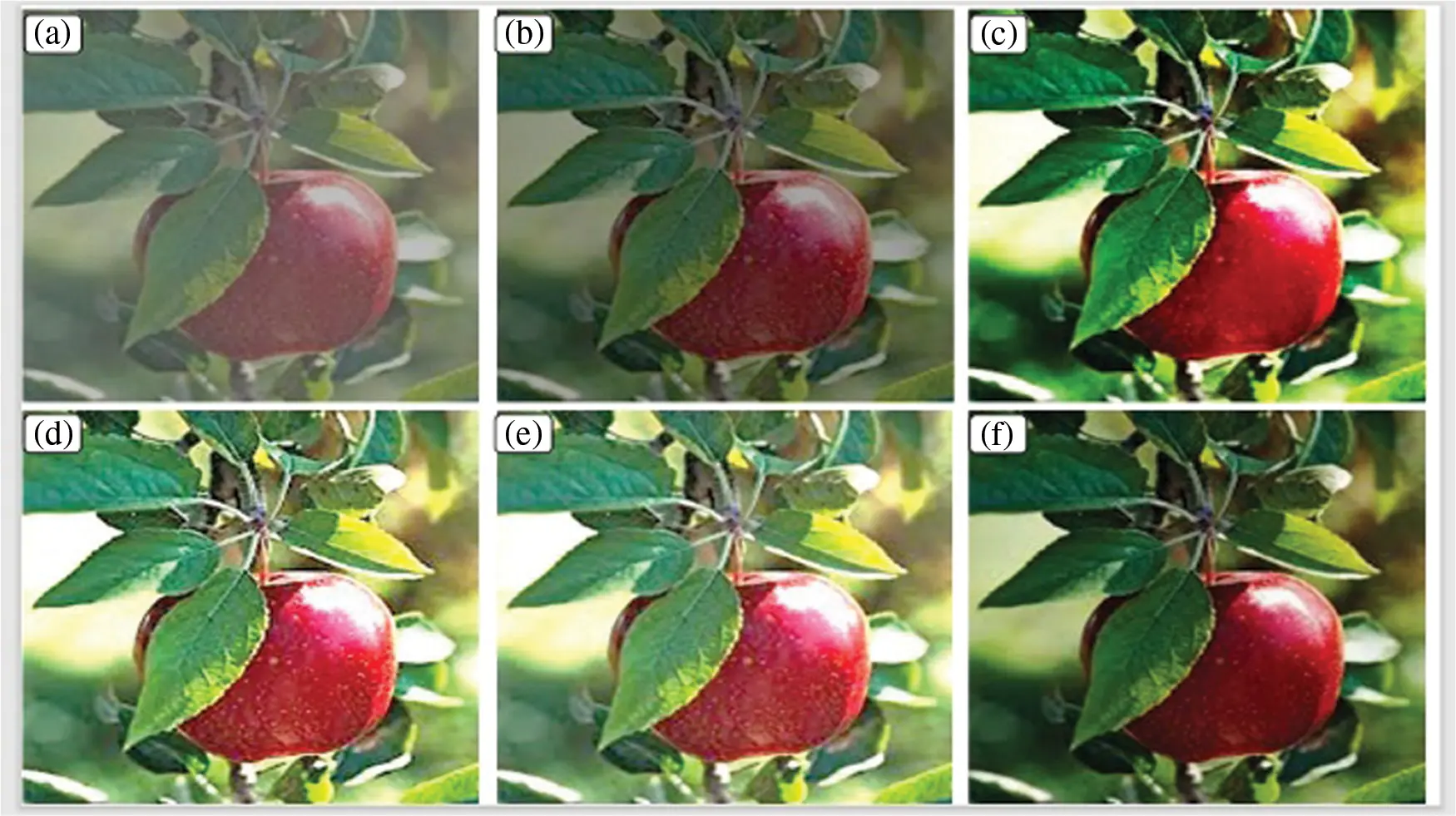Figure 1: Fruit image visual analysis  (a) input image, (b) (b) enhanced by ATCE, (C) enhanced by BPDHE, (d) enhanced by GC, and (e) by CLAHE, and (f) by Type-II Fuzzy

4  Proposed Deep Learning Model

In the proposed approach, the major objective is to extract the optimal features, label the optimal features and finally classify the fruits based on optimal features by deep learning applications. In this research work, CNN, RNN, and LSTM deep learning models are combined to classify the fruits. During feature extraction using CNN, segment acquired features into different strategies, we divide the features into coarse and fine categories. CNN generator is involved to extract fine and coarse label categories. The two layers can be structured either in a sequential or parallel way. The general architect of CNN is as follows:

IMAGE[(CONVRELU)NCRPOOL]NCRPFC(29)

IMAGE: Input Image

CONV: Convolutional Layer

RELU: Rectified Linear Unit

POOL: Pooling Layer

NCR: up to 5

NCRP is Large

FC: Contains neurons that connect to the entire input volume, as in ordinary Neural Networks.

Let I will be input image, then convolution operation is defined as:

Cp=RELU(IKp+bp)(30)

where, kernel Kp is applied on input image I and bp are biased for pth convolution image.

RELU is a linear activation function that is similar to a filter that allows positive inputs to pass through unchanged while clamping everything else to zero. It is defined as

RELU(x)={0x0xx>0(31)

Let Sp (x, y) be pth sampling image after applying pooling operation on activation map Cp. When filter size m×n = 2 × 2, no padding (P = 0) and stride (s = 2), then AVG pooling is defined as follows

Sp1(x,y)=14u=01v=01Cp1(2xu,2yv)(32)

This work is done by the approach used by Guo et al. to label the image features, we employed the same concept to label the fruit image features as shown in Fig. 5. Four different strategies are designed to divide the features. Coarse to fine, fine to coarse, fine and coarse, and finally RNN. Here RNN is involved because CNN is unable to label the extracted features effectively.

During the categorization policy, firstly we separate training and testing data into 75% and 25%, Then 25% into training, and 75% into testing. Features are extracted but not categorized into different labels efficiently. Therefore, we employed RNN to label the features. The Convolution Neural Network-based developer is unable to exploit the interrelationship between two supervisory signals individually. In case, if the hierarchy has adaptive size, a CNN-based developer is not able to categorize the hierarchical labels. To overcome the limitations of CNN, the RNN-LSTM integrator calculates hierarchical classification by altering the last layer of a CNN with RNN.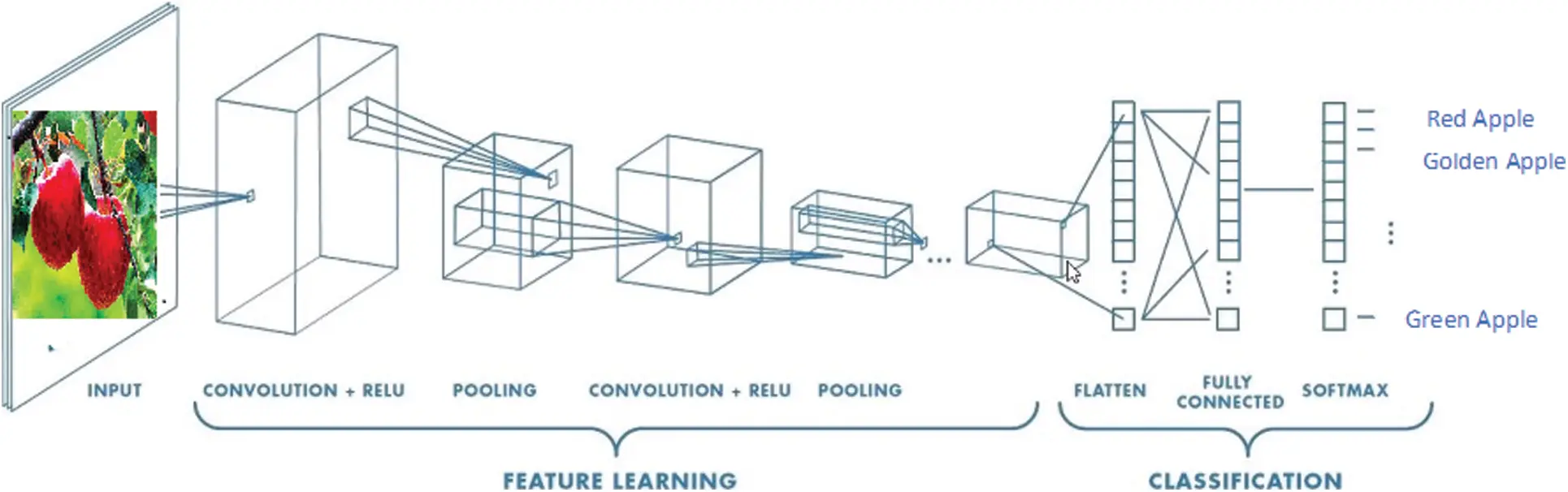Figure 2: CNN image extraction model

4.1 RNN-LSTM Integration

RNN is an artificial neural network in which edges between units form a directed cycle (see Fig. 4). RNN model dynamic classification behavior effectively but unable to develop long-term labeling modeling. LSTM is integrated with RNN to model the dynamic labeling impressively. Therefore, Long-Short Term Memory (LSTM) is used in this paper to overcome the issues with RNN.Figure 3: Fine and coarse label strategy [3,24]

During the Proposed classification scheme, the major objective of the study is to classify the fruit images quite impressively. We integrate the RNN and CNN to overcome the limitations of CNN to label and classify the fruits. The flow is depicted in Fig. 5.

As shown in Fig. 4, features extracted and labeled using CNN and RNN respectively are fed into LSTM for deep learning fruit classification. softmax, fully connected and classification layers are employed to find and label the input fruit images. To train the fruit image classification model, by considering the ground truth, coarse and fine labels are predicted as:

Loss=1Ni=1N(t=1Tj=1C+F1{xti=j}logpj)(33)Figure 4: RNN (left) and LSTM (right) collaboration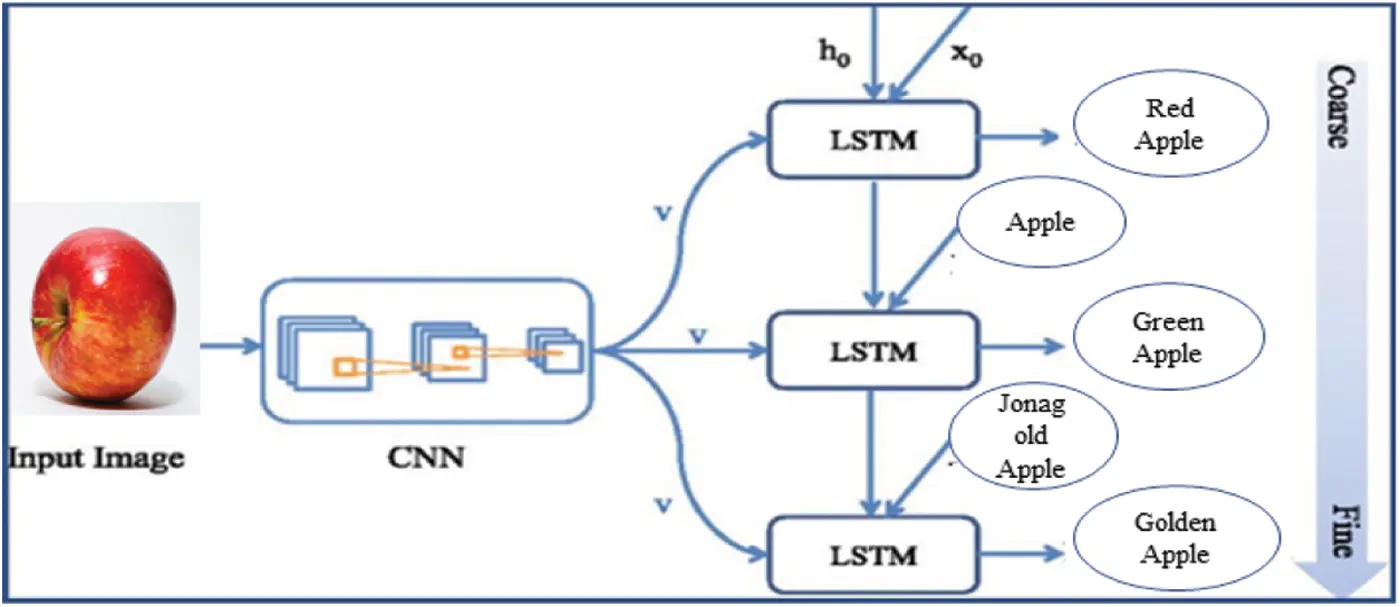Figure 5: The integrated framework 

During the inference phase, the CNN-RNN integrator predicts the probability labeling for the current timestamp when the ground truth coarse-fine strategy is not available. To overcome the CNN-RNN limitations, LSTM is used to label the optimal and effective features during image classification.

4.2 Deep Learning Fruit Classification Model

In the proposed deep learning model CNN, RNN and LSTM were used to classify the fruits. In the proposed model, extracted features using the convolution layer of CNN are labeled are coarse and fine with the help of RNN. Linear, convolution and fully connected layers of CNN extract the best-matched feature based on intensity, texture, and shape. RNN is involved to label these features distinctly. Finally soft-max-layer are used to classify the fruits by the filtering process used by LSTM. Optimal features are utilized in the proposed approach to classifying the fruits.

As shown in Fig. 6, a collaboration of three deep learning applications is generated. The proposed classification approach trains the fruit classification model using the effective features. CNN uses linear, convolution, and fully connected layers to extract the image features. After successfully extracting the image features, RNN handles the feature labeling strategy quite efficiently. Extracted and labeled features are fed into LSTM using best-matched features. Image filter strategy is used during the LSTM approach to classifying the fruits.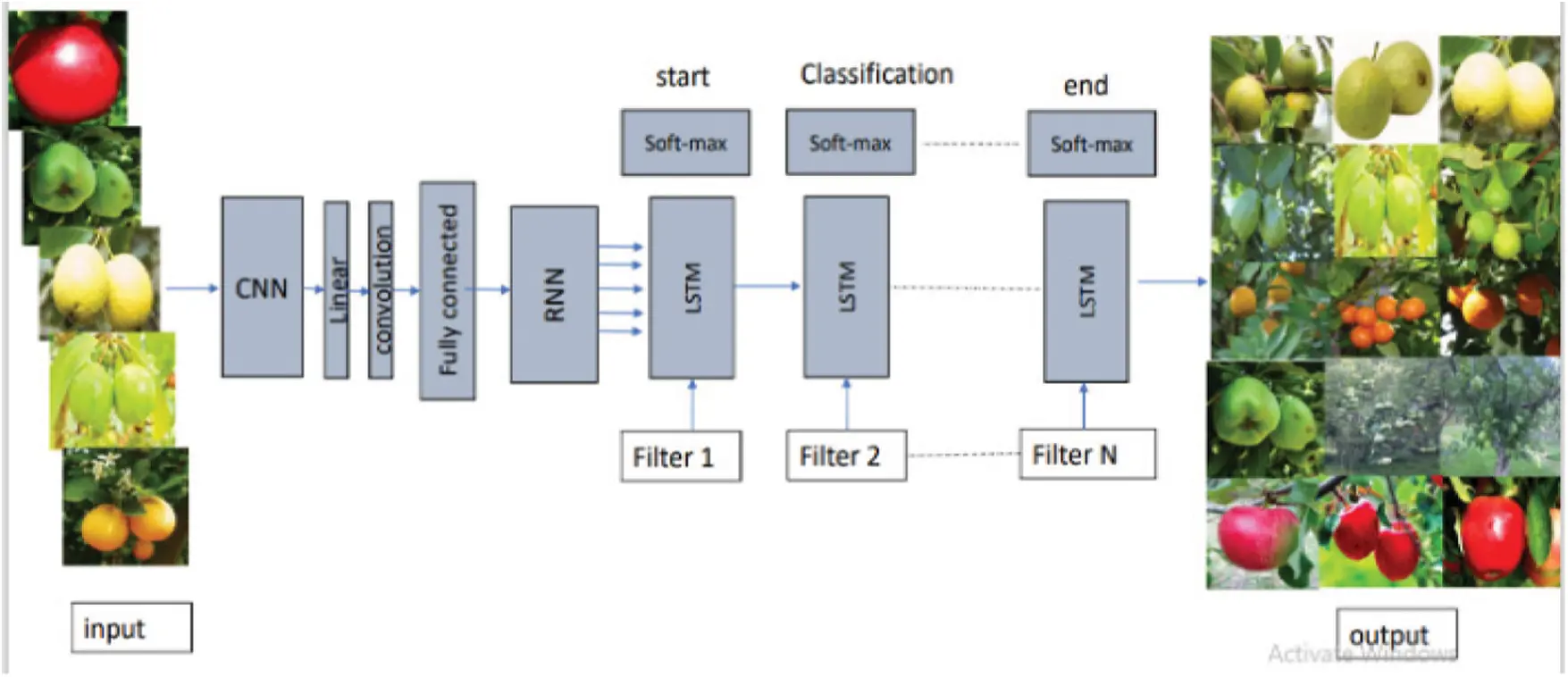Figure 6: Proposed fruit classification model

5  Results and Discussion

To evaluate the classification performance of the proposed scheme, MATLAB software is used for simulation work on the Intel Core i5 processor with 8−GB RAM. Deep learning applications CNN, RNN, and LSTM are integrated to develop a novel fruit image classification model. SVM, FFNN, and ANFIS classification results are compared with the proposed scheme.

In the proposed study accuracy, root means square, and coefficient of correlation analysis measures are employed to evaluate the performance of the novel fruit image classification approach.

5.1 Accuracy Analysis

Few 1's determine the quality of fruit images during classification. In the same way, 0's described the failure rate. Classification accuracy is defined as:

Accuracy=TP+TNTP+TN+FP+FN(34)

Here Tp+Tn+Fp+Fn represent true positive, true negative, false positive, and false negative, correspondingly.

Fig. 7 depicts the accuracy analysis of the proposed and existing SVM, FFNN, ANFIS, and RNN-CNN fruit classification techniques. The proposed approach accuracy rate is better in comparison with the competitive approaches.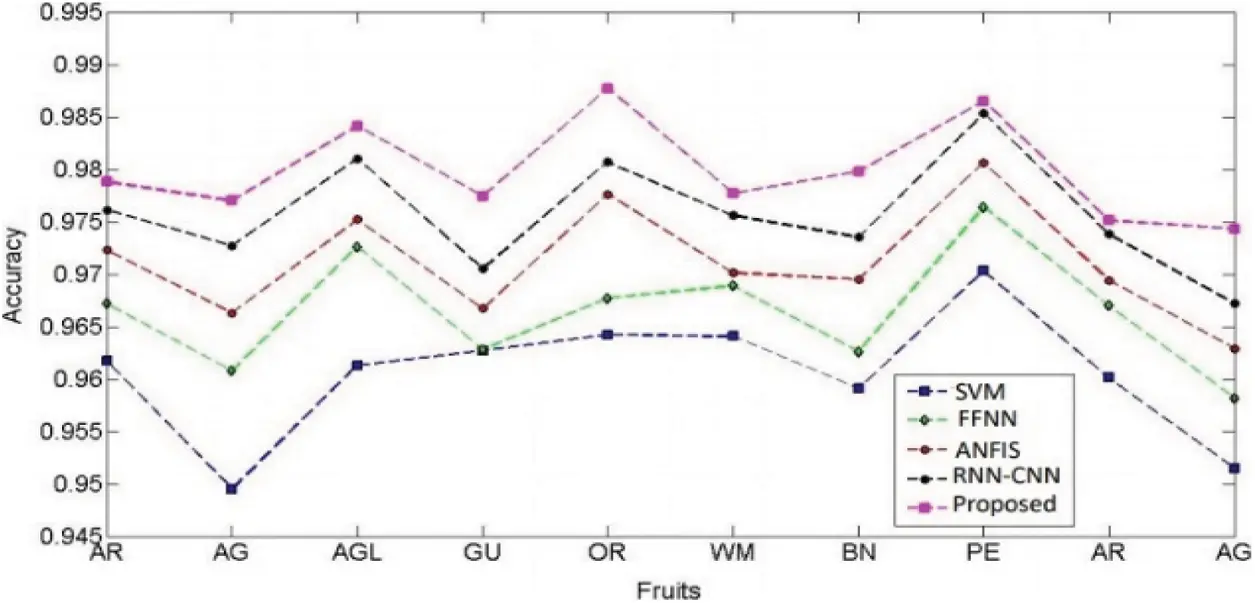Figure 7: Accuracy analysis

5.2 F-Measure

F-measure is used to evaluate the accuracy of the proposed classification scheme as shown in Fig. 8. F-measure consists of p(precision) and r(recall). Matching positive results are defined by p and dividing the number of all positive results by r, which is the number of all positive matching results. F-measure is harmonic mean for all p and r values.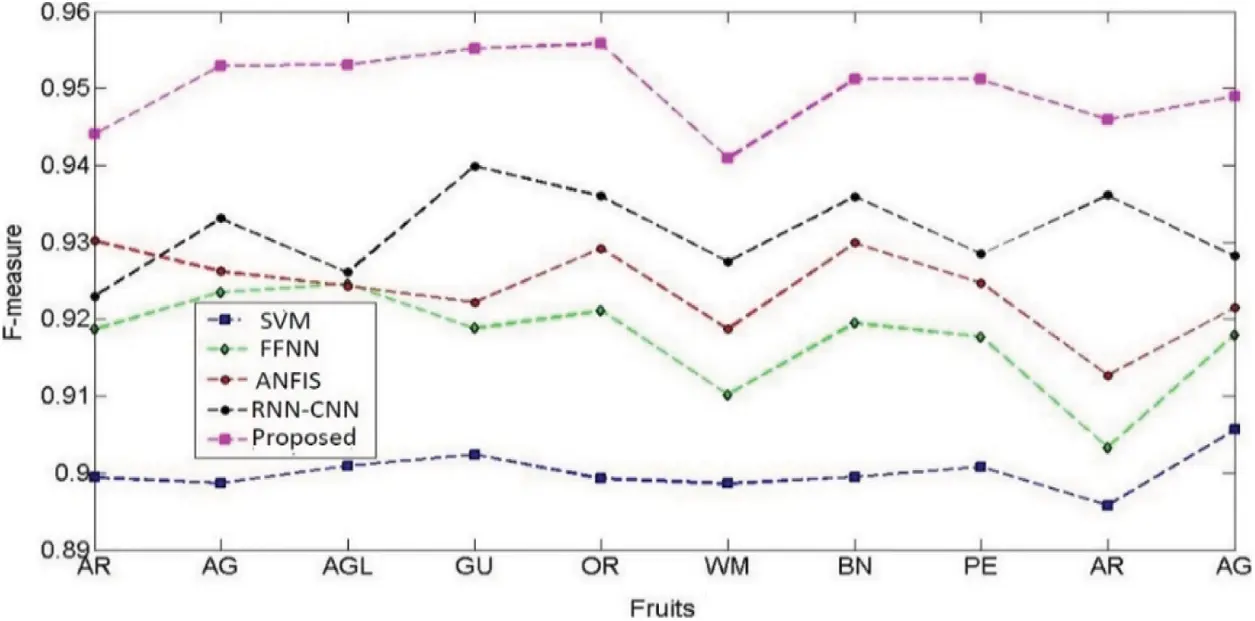Figure 8: F-measure

Here 1 value for F measure means best classifier result and 0 means worst classification results. Precision is the fraction of relevant values among the obtained values and recall is the fraction of relevant values that have been obtained from the total obtained classification values. Precise is positive value and recall is called sensitivity during the classification process.

F-measure is defined as:

Fmeasure=2×Precision×RecallPrecision+Recall(35)

Precision and recall are defined as:

Precision=TPTP+FP(36)

Recall=TPTP+FN(37)

5.3 Discussion

Deep Learning models are proposed for fruit image classification. Each image of size 512 × 512 is utilized with the help of MATLAB software for classification purposes. The preliminary step of the proposed study is to enhance the fruit images. Type-II fuzzy is implemented in the proposed scheme. The results of the proposed method are compared with existing competitive methods. Edges are preserved, low pixel saturation during fruit image enhancement by Type-II Fuzzy. The proposed approach successfully solves the problem of classification issues, that occur during the state of art approaches.

Three objectives were achieved, CNN is used to meet the first objective of feature extraction. RNN is involved to meet the labeling of optimal features objective. LSTM is finally used to meet the image classification objectives. The results of this study are compared with the existing classification approaches. The results of SVM are sufficient to meet the standards but fail to provide accurate outcomes during texture features classifications, which are satisfactory by the proposed approach. Results of FFNN are also quite promising but fail in comparison to true positive and true negative analysis, which are better during the proposed classifier. ANFIS has good accuracy rates but fails to match the results of the proposed scheme during f measure quantitative analysis. combination of RNN-CNN has a better classification rate but is inadequate during classification after feature extraction and labeling procedure.

6  Conclusion

Due to their similar form but the varied texture and intensity characteristics, fruit categorization remains a difficult job. We developed a deep learning method in this paper to classify the fruit images. To create discriminative features, CNN was employed, while RNN was used to generate sequential labels. Based on optimum and labeled features by CNN and RNN, LSTM was used to categorize the fruit images extremely well. On fruit images, extensive experiments were conducted utilizing the suggested method as well as current classification algorithms such as SVM, FFNN, and ANFIS. In terms of accuracy, root means square, and coefficient of correlation analysis, this classification method surpasses current image classification algorithms. This work has been done on the acquired fruit images, which may have limitations in the form of contrast improvement and edge detection. In the future, work be elaborated to overcome these issues.

Acknowledgement: We deeply acknowledge Taif University for supporting this study through Taif University Researchers Supporting Project Number (TURSP-2020/150), Taif University, Taif, Saudi Arabia.

Funding Statement: This research is funded by Taif University, TURSP-2020/150.

Conflicts of Interest: The authors declare that they have no conflicts of interest to report regarding the present study.

## References

1. L. Zheng, H. Shi and M. Gu, “Infrared traffic image enhancement algorithm based on dark channel prior and gamma correction,” Modern Physics Letters B, vol. 31, no. 19–21, pp. 174004401–174004407, 2017.
2. D. Singh and V. Kumar, “Comprehensive survey on haze removal techniques,” Multimedia Tools and Applications, vol. 77, pp. 9595–9620, 2017.
3. H. S. Gill and B. S. Khehra, “Efficient image classification technique for weather degraded fruit images,” IET Image Processing, vol. 14, no. 14, pp. 3463–3470, 2020.
4. J. Lu, J. Hu, G. Zhao, F. Mei and C. Zhang, “An in-field automatic wheat disease diagnosis system,” Computers and Electronics in Agriculture, vol. 142, pp. 369–379, 2017.
5. T. T. Le and C. Y. Lin, “Deep learning for noninvasive classification of clustered horticultural crops–A case for banana fruit tiers,” Postharvest Biology and Technology, vol. 156, pp. 110922, 2019.
6. M. M. Sofu, E. Orhan, M. C. Kayacan and B. Cetişli, Design of an automatic apple sorting system using machine vision,” Computers and Electronics in Agriculture, vol. 127, pp. 395–405, 201
7. Y. Guo, Y. Liu, E. M. Bakker, Y. Guo and M. S. Lew, “Cnn-rnn: A large-scale hierarchical image classification framework,” Multimedia Tools and Applications, vol. 77, pp. 10251–10271, 2018.
8. Y. Zhang and L. Wu, “Classification of fruits using computer vision and a multicast support vector machine,” Sensors, vol. 12, no. 9, pp. 12489–12505, 2012.
9. M. S. Hossain and M. Ah-Hammadi, “Automatic fruit classification using deep learning for industrial applications,” IEEE Transactions on Industrial Informatics, vol. 15, no. 2, pp. 1027–1034, 2018.
10. Z. M. Khaing, Y. Naung and P. H. Tut, “Development of control system for fruit classification based on convolutional neural network,” in IEEE Conf. of Russian Young Researchers in Electrical and Electronic Engineering (EIConRus), St. Petersburg and Moscow, Russia, 2018.
11. H. Deng, X. Sun, M. Liu, C. Ye and X. Zhou, “Image enhancement based on intuitionistic fuzzy sets theory,” IET Image Processing, vol. 10, no. 10, pp. 701–709, 2016.
12. M. Horea and M. Oltean. “Fruit recognition from images using deep learning,” Computer Vision and Pattern Recognition, vol. 17, no. 12, pp. 576–580, 2017.
13. R. Aranda, N. Varela, C. Tello and R. G. Ramirez, “Fruit Classification for Retail Stores Using Deep Learning,” in Mexican Conference on Pattern Recognition, Springer, Cham, pp. 3–13, 2020.
14. A. T. Nasiri. A. Garavand and Y. D. Zhang, “Image-based deep learning automated sorting of date fruit,” Postharvest Biology and Technology, vol. 153, pp. 133–141, 2019.
15. M. Turkoglu, D. Hanbay and A. Sengur, “Multi-model lstm-based convolution neural networks for detection of apple diseases and pests,” Journal of Ambient Intelligence and Humanized Computing, vol. 22, pp. 1–11, 2019.
16. Y. D. Zhang, Z. Dong, X. Chen, W. Jia, S. Du et al., “Image-based fruit category classification by 13-layer deep convolutional neural network and data augmentation,” Multimedia Tools and Applications, vol. 78, no. 3, pp. 3613–3632, 2019.
17. A. A. Sen, N. M. Bahbouh, A. B. Alkhodre, A. M. Aldhawi, F. A. Aldham et al., “A classification algorithm for date fruits,” in 7th IEEE Int. Conf. on Computing for Sustainable Global Development (INDIACom), pp. 235–239, 2020.
18. A. A. Akif and M. F. Khan, “Automatic classification of plants based on their leaves,” Biosystems Engineering, vol. 139, pp. 66–75, 2015.
19. S. Benalia, S. Cubero, J. M. Montalb, B. Bernardi, G. Zimbalatti et al., “Computer vision for automatic quality inspection of dried figs (ficus carica) in real-time,” Computers and Electronics in Agriculture, vol. 120, pp. 17–25, 2016.
20. A. K. Rangarajan, R. Purushothaman and A. Ramesh, “Tomato crop disease classification using pre-trained deep learning algorithm,” Procedia Computer Science, vol. 133, pp. 1040–1047, 2018.
21. Y. Song, C. Glasbey, G. Horgan, G. Polder, J. Dieleman et al., “Automatic fruit recognition and counting from multiple images,” Biosystems Engineering, vol. 118, pp. 203–215, 2014.
22. H. Chaudhry, M. S. M. Rahim and A. Khalid, “Multi scale entropy-based adaptive fuzzy contrast image enhancement for crowd images,” Multimedia Tools and Applications, vol. 77, pp. 15485–15504, 2017.
23. A. F. Zadeh, “Fuzzy logic,” Computer, vol. 21, no. 4, pp. 83–93, 1988.
24. H. Singh and B. S. khehra, “Visibility enhancement of color images using type-II fuzzy membership function,” Modern Physics Letters B, vol. 32, no. 11, pp. 185501301–185513014, 2018.
25. C. Dang, J. Gao, Z. Wang, F. Chen and Y. Xiao, “Multi-step radiographic image enhancement conforming to weld defect segmentation,” IET Image Processing, vol. 9, no. 11, pp. 943–950, 2015.
26. A. Naji, S. H. Lee and J. Chahl, “Quality index evaluation of videos based on fuzzy interface system,” IET Image Processing, vol. 11, no. 5, pp. 292–300, 2017.
27. F. D. Mu, Z. Deng and J. Yan, “Uncovering the fuzzy community structure accurately based on steepest descent projection,” Modern Physics Letters B, vol. 31, no. 27, pp. 1750249, 2017.
28. Z. Tian, L. M. Jia, H. H. Dong, Z. D. Zhang and Y. D. Ye, “Fuzzy peak hour for urban road traffic network,” Modern Physics Letters B, vol. 29, no. 15, pp. 1550074, 2015.
29. W. J. Yoo, D. H. Ji and S. C. Won, “Adaptive fuzzy synchronization of two different chaotic systems with stochastic unknown parameters,” Modern Physics Letters B, vol. 24, no. 10, pp. 979–994, 2010.
30. Y. Tang and J. A. Fang, “Synchronization of takagi-sugeno fuzzy stochastic delayed complex networks with hybrid coupling,” Modern Physics Letters B, vol. 23, no. 21, pp. 2429–2447, 2009.
31. M. Andrecut, “A Statistical-fuzzy perceptron,” Modern Physics Letters B, vol. 13, no. 1, pp. 33–41, 1999.
32. J. P. Shi, “Prediction study on the degeneration of lithium-ion battery based on fuzzy inference system,” Modern Physics Letters B, vol. 31, no. 19–21, pp. 1740083, 2017.
33. C. Dong, C. C. Loy, K. He and X. Tang, “Image super-resolution using deep convolutional networks,” IEEE Transactions on Pattern Analysis and Machine Intelligence, vol. 38, no. 2, pp. 295–307, 2016.
34. K. Singh, D. K. Vishwakarma. G. S. Walia and R. Kapoor, “Contrast enhancement via texture region-based histogram equalization,” Journal of Modern Optics, vol. 63, no. 15, pp. 1444–1450, 2016.
35. H. Dong and M. Liu, “Image enhancement based on intuitionistic fuzzy set entropy,” IET Image Processing, 2016, vol. 10, no. 10, pp. 701–701.
36. H. S. Gill and B. S. Khehra, “Hybrid classifier model for fruit classification,” Multimedia Tools and Applications, vol. 80, pp. 11042–11077, 2021.
37. Y. Song, C. A. Glasbey, G. W. Horgan, G. Polder, J. A. Dieleman et al., “Automatic fruit recognition and counting from multiple images,” Biosystems Engineering, vol. 118, pp. 203–215, 2014.This work is licensed under a Creative Commons Attribution 4.0 International License, which permits unrestricted use, distribution, and reproduction in any medium, provided the original work is properly cited.